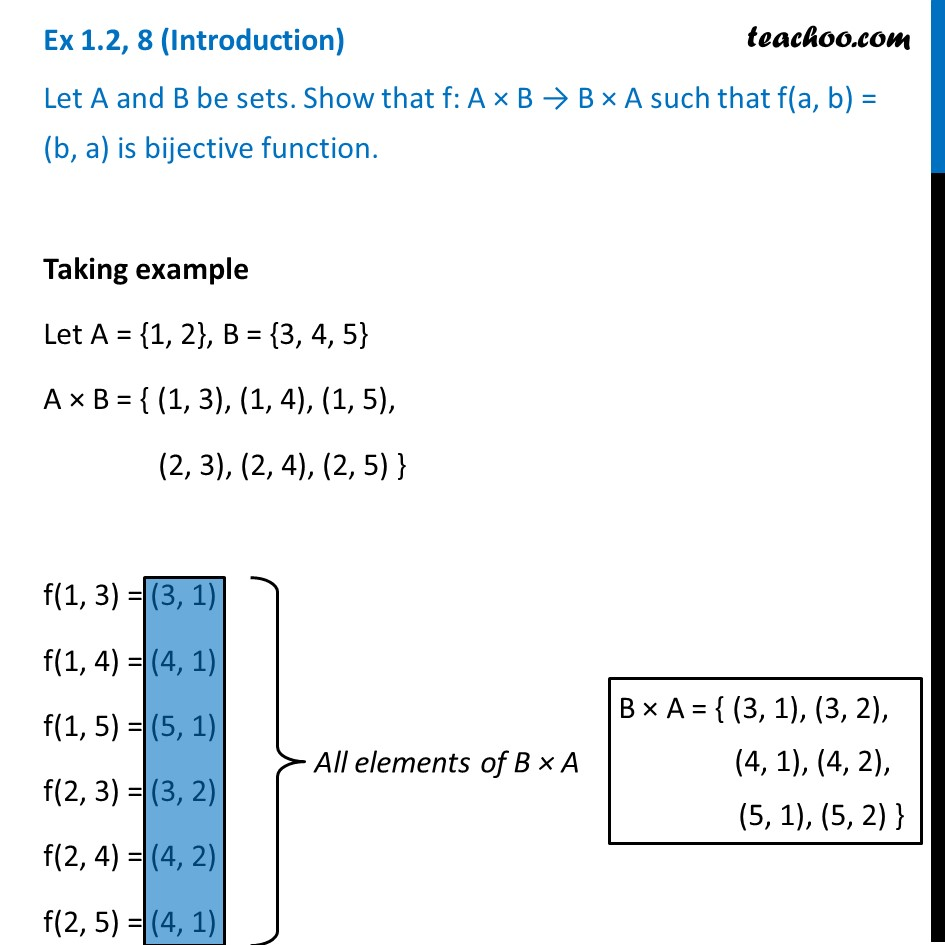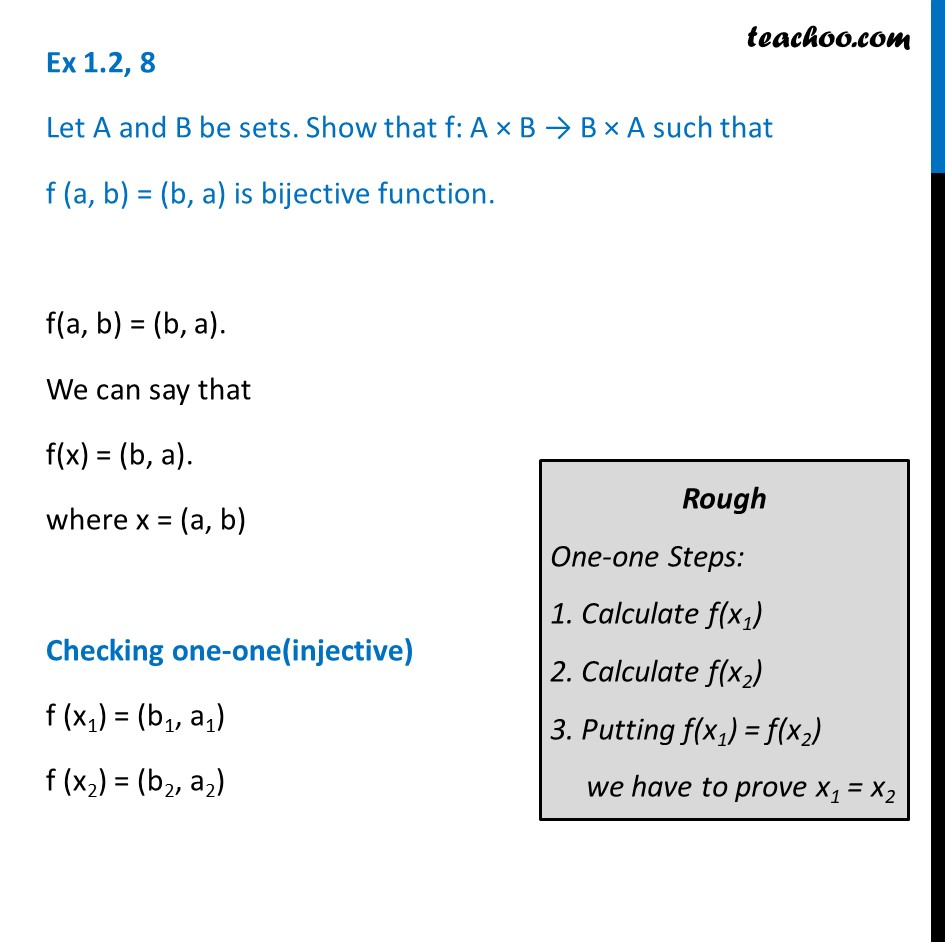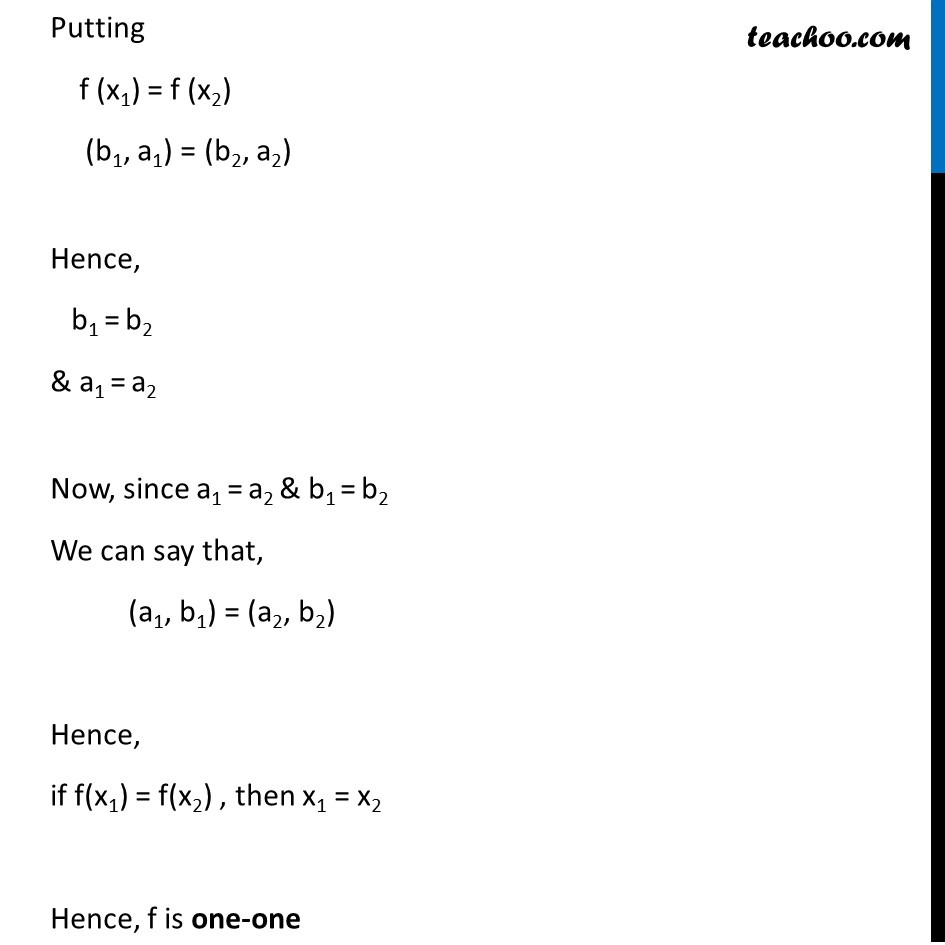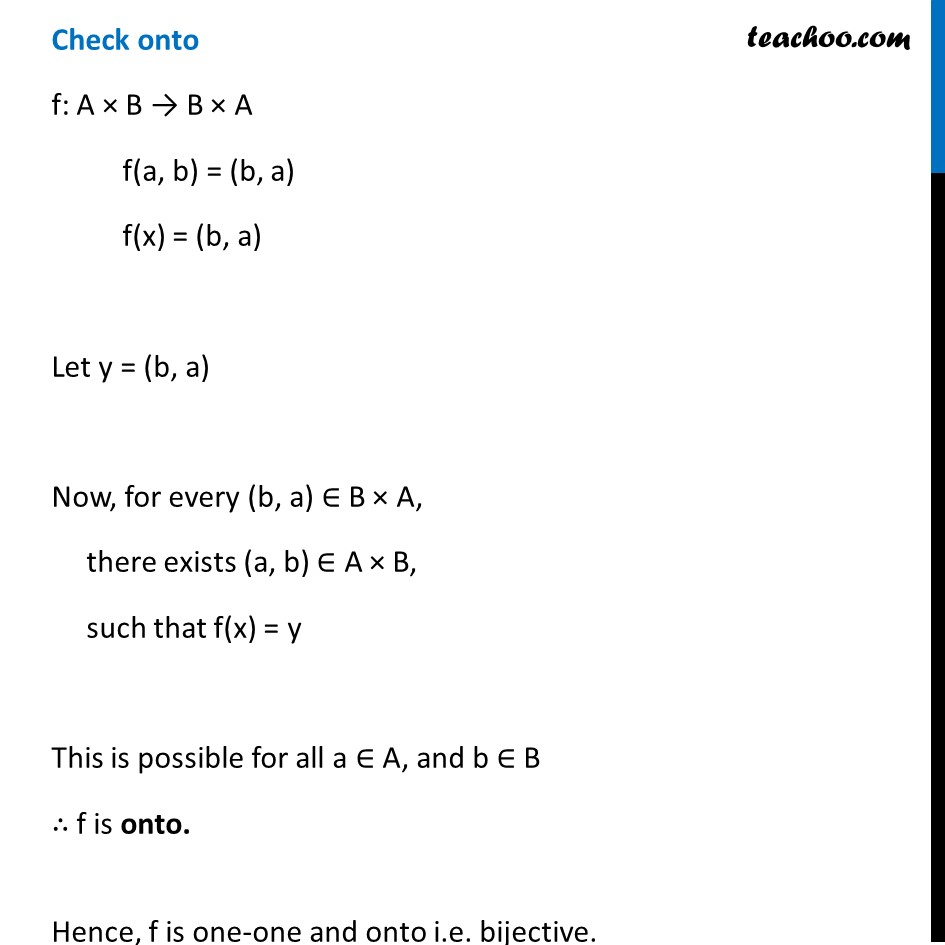To prove one-one & onto (injective, surjective, bijective)

Chapter 1 Class 12 Relation and Functions
Concept wiseLearn in your speed, with individual attention - Teachoo Maths 1-on-1 Class

### Transcript

Ex 1.2, 8 (Introduction) Let A and B be sets. Show that f: A × B → B × A such that f(a, b) = (b, a) is bijective function. Taking example Let A = {1, 2}, B = {3, 4, 5} A × B = { (1, 3), (1, 4), (1, 5), (2, 3), (2, 4), (2, 5) } f(1, 3) = (3, 1) f(1, 4) = (4, 1) f(1, 5) = (5, 1) f(2, 3) = (3, 2) f(2, 4) = (4, 2) f(2, 5) = (4, 1) All elements of B × A B × A = { (3, 1), (3, 2), (4, 1), (4, 2), (5, 1), (5, 2) } Ex 1.2, 8 Let A and B be sets. Show that f: A × B → B × A such that f (a, b) = (b, a) is bijective function. f(a, b) = (b, a). We can say that f(x) = (b, a). where x = (a, b) Checking one-one(injective) f (x1) = (b1, a1) f (x2) = (b2, a2) Rough One-one Steps: 1. Calculate f(x1) 2. Calculate f(x2) 3. Putting f(x1) = f(x2) we have to prove x1 = x2 Putting f (x1) = f (x2) (b1, a1) = (b2, a2) Hence, b1 = b2 & a1 = a2 Now, since a1 = a2 & b1 = b2 We can say that, (a1, b1) = (a2, b2) Hence, if f(x1) = f(x2) , then x1 = x2 Hence, f is one-one Check onto f: A × B → B × A f(a, b) = (b, a) f(x) = (b, a) Let y = (b, a) Now, for every (b, a) ∈ B × A, there exists (a, b) ∈ A × B, such that f(x) = y This is possible for all a ∈ A, and b ∈ B ∴ f is onto. Hence, f is one-one and onto i.e. bijective.